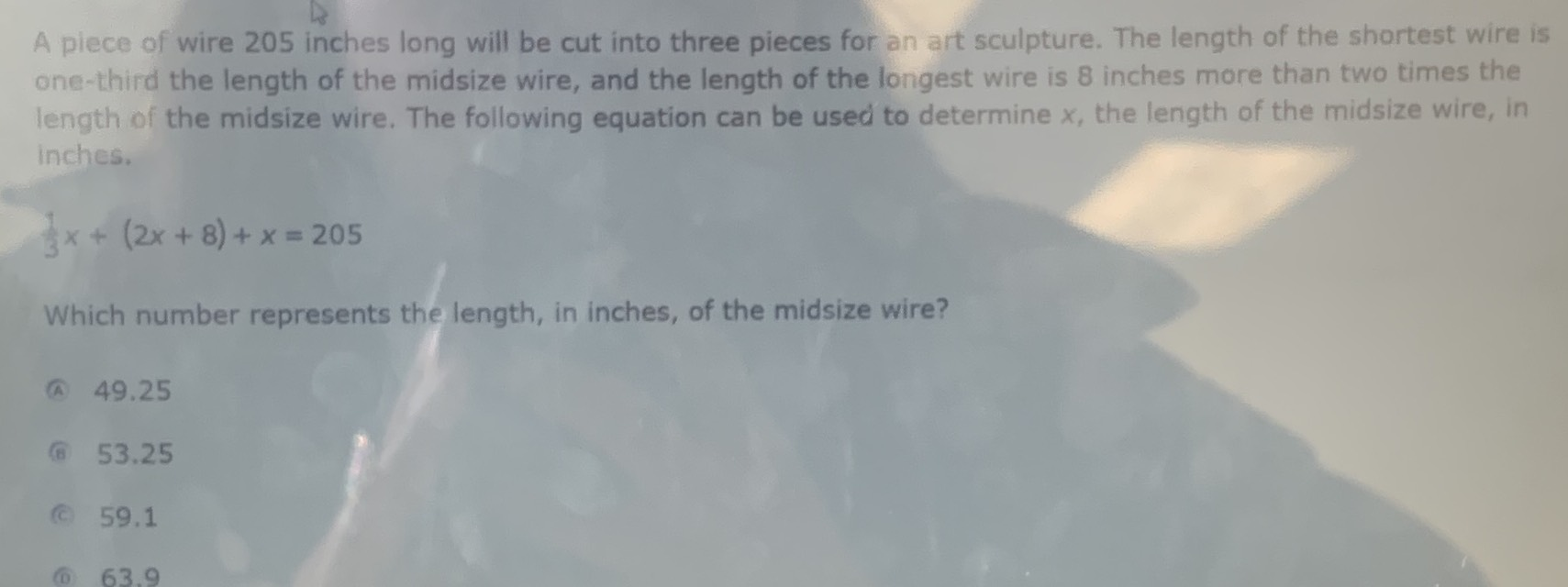### ¿Todavía tienes preguntas de matemáticas?

Pregunte a nuestros tutores expertos
Algebra
PreguntaA piece of wire $$205$$ inches long will be cut into three pieces for an art sculpture. The length of the shortest wire is one-third the length of the midsize wire, and the length of the longest wire is $$8$$ inches more than two times the length of the midsize wire. The following equation can be used to determine $$x$$ , the length of the midsize wire, in inches.

$$\frac { 1 } { 3 } x + ( 2 x + 8 ) + x = 205$$

Which number represents the length, in inches, of the midsize wire?

(A) $$49.25$$     (B) $$53.25$$      (C) $$59.1$$            (D) $$63.9$$